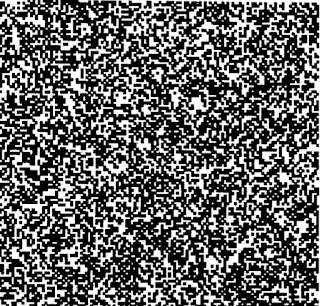## Wednesday, 21 July 2021

### A New Matrix Mathematics for Deep Learning : Random Matrix Theory of Deep Learning

PreambleFigure: Definition of Randomness (Compagner 1991, Delft University)
Development of deep learning systems (DLs)  increased our hopes to develop more autonomous systems. Based on the hierarchal learning of representations, deep learning defies the basic learning theory that beg the question of still rethinking generalisation. Even though DLs lacks severely the ability to reason without causal inference, they can't do that in vanilla form. However despite this limitation, they provide very rich new mathematical concepts as introduced recently. Here, we review couple of these new concepts briefly and draw attention to Random Matrix Theory's relevance in DLs and its applications in Brain networks.  These concepts in isolation are subject of applied mathematics but their interpretation and usage in deep learning architectures are demonstrated recently. In this post we provide a glossary of new concepts, that are not only theoretically interesting, they are directly practical from measuring architecture complexity to equivalance.

Random matrices can simulate deep learning architectures with spectral ergodicity

Random Matrix Theory (RMT) has origins in foundation of mathematical statistics and mathematical physics pioneered by Wishart Distribution and Dyson Circular Ensembles.  As primary ingredient of a deep learning model as a result are set of weights, or learned parameter set, manifests as matrices and they come from a learning dynamics that are used in so called in inference time. Natural consequence of this, learning these matrices can be simulated via Random matrices of spectral radius close to unity. This provides us the following, ability to make a generic statement about deep learning systems independent of
1. Network architecture (topology).
2. Learning algorithm.
3. Data sizes and type.
4. Training procedure.

Why not Hessian or loss-landscape but Weight matrices?

There are studies taking Hessian matrix as a major object, i.e., second derivative of parameters as a function of loss of the network and associate this to random matrices. However, this approach would only covers learning algorithm properties rather than architectures inference or learning capacity. For this reason, weight matrices should be taken as a primary object in any studies of random matrix theory in deep learning as they encode depth in deep learning. Similarly, loss-landscape can not capture the capacity of deep learning.

Conclusion and outlook

In this short exposition, we tried to stimulate readers interest in exciting set of tools from RMTs for deep learning theory and practice. That is still subject of recent research with direct practical relevance. We provided glossary and reading list as well.

Papers introducing new mathematical concepts in deep learning are listed here, they come with associated Python codes for reproducing the concepts.

Earlier relevant blog posts

Citing this post

A New Matrix Mathematics of Deep Learning: Random Matrix Theory of Deep Learning : https://science-memo.blogspot.com/2021/07/random-matrix-theory-deep-learning.html Mehmet Süzen, 2021

Glossary of New Mathematical Concepts of Deep Learning

Summary of the definition of new mathematical concepts for new matrix mathematics.

Spectral Ergodicity Measure of ergodicity in spectra of a given random matrix ensemble sizes. Given set of matrices of equal size that are coming from the same ensemble, average deviation of spectral densities of individual eigenvalues over ensemble averaged eigenvalue. This mimic standard ergodicity, instead of over states of the observable, it measures ergodicity over eigenvalue densities.  $\Omega_{k}^{N}$, $k$-th eigenvalue and matrix size of $N$.

Spectral Ergodicity Distance A symmetric distance constructed with two Kullback-Leibler distances over two different size matrix ensembles, in two different direction. $D = KL(N_{a}|N_{b})+ KL(N_{b}|N_{a})$

Mixed Random Matrix Ensemble (MME) Set of matrices constructed from a random ensemble but with difference matrix sizes from N to 2, sizes determined randomly with a coefficient of mixture.

Periodic Spectral Ergodicity (PSE) A measure of Spectral ergodicity for MMEs whereby smaller matrix spectrum placed in periodic boundary conditions, i.e., cyclic list of eigenvalues, simply repeating them up to N eigenvalues.

Layer Matrices Set of learned weight matrices up to a layer in deep learning architecture. Convolutional layers mapped into a matrix, i.e. stacked up.

Cascading Periodic Spectral Ergodicity (cPSE) Measuring PSE over feedforward manner in a deep neural network.  Ensemble size is taken up-to that layer matrices.

Circular Spectral Deviation (CSD) This is a measure of fluctuations in spectral density between two ensembles.

Matrix Ensemble Equivalence If CSDs are vanishing for conjugate MMEs, they are said to be equivalent.

Appendix: Practical Python Example

Complexity measure for deep architectures and random matrix ensembles: cPSE.cpse_measure_vanilla Python package Bristol  (>= v0.2.12) has now a support for computing cPSE from a list of matrices, no need to put things in torch model format by default.

!pip install bristol==0.2.12

An example case:

from bristol import cPSE

import numpy as np

np.random.seed(42)

matrices = [np.random.normal(size=(64,64)) for _ in range(10)]

(d_layers, cpse) = cPSE.cpse_measure_vanilla(matrices)

d_layers is decreasing vector, it will saturate at some point, that point is where adding more

layers won’t improve the performance. This is data, learning or architecture independent measure.

Only a French word can explain the excitement here: Voilà!